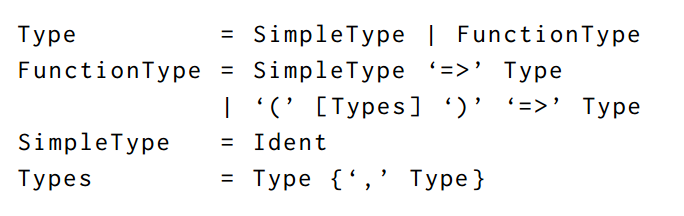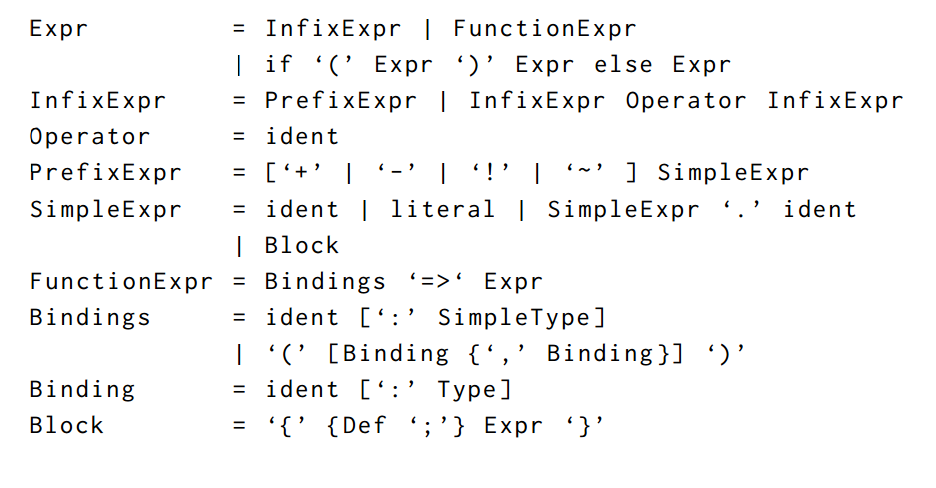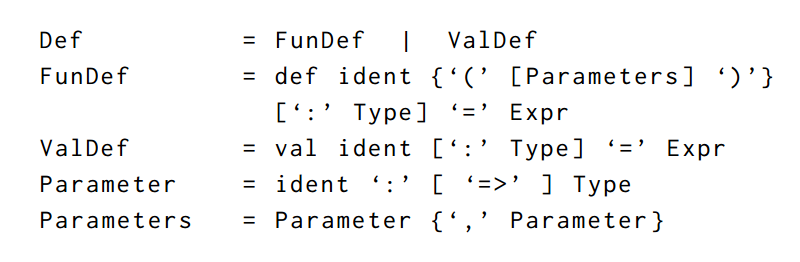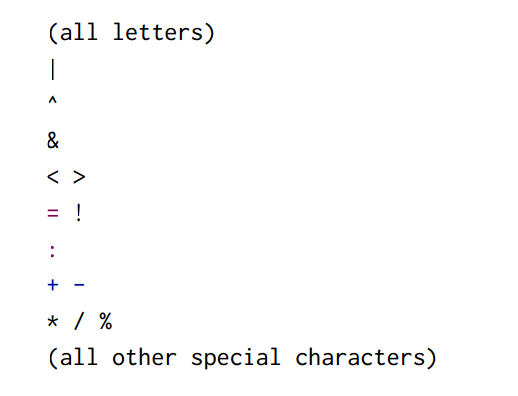匿名函数

{ def f(x1 : T1, …, xn : Tn) = E; f }

Scala 语法汇总

• | ：替代关系
• [...] 0 或 1 个
• {...} 0 或 多个

类型（Types）• 数字：Int、Double（Byte、Short、Char、Long、Float）
• 布尔：true 或 false
• 字符串
• 函数：像是 Int => Int 或者 (Int, Int) => Int

表达式（Expressions）• 标识符：例如 x 或是 isGoodEnough
• 常量：例如 01.0 或是 "abc"
• 执行函数：例如 sqrt(x)
• 执行运算符：例如 -xx + y
• 选择表达式：例如 math.abs （这里不太懂 selection是指的什么，该方法的内部实现是用的选择表达式？）
• 条件表达式：例如 if (x < 0) -x else x
• 代码块：例如 { val x = math.abs(y) ; x * 2 }
• 匿名函数：例如 x => x + 1

定义（Definitions）• 方法定义：例如 def square(x: Int) = x
• 值定义：例如 val y = square(2)

• 值调用：例如 (x: Int)
• 名称调用：例如 (y: => Double)

函数和数据

类

1. 一个新的类型（Type）：Rational
2. 一个可以用于创建 Rational 实例的构造方法（Constructor）

Scala 会保证定义的名称和值在不同的命名空间（Namespace）中，所以多个 Rational 定义彼此之间不会冲突（？）

前提检验

require 是一个预定义方法，它需要一个条件以及可选的提示信息。当条件为假时，将会抛出一个携带提示信息的 IllegalArgumentException 异常。

断言

• require 适合在方法执行前校验外部传入的参数
• assert 用于校验方法执行过程中的逻辑

• 接收传入的参数
• 执行类体中的所有语句

类中的代换模型

• w1, ..., wn 被代换为了方法 f 的形参 y1, ..., yn
• v1, ..., vn 被代换为了类 C 的形参 x1, ..., xm
• 表达式 new C(v1, ..., vn) 被代换为了自引用 this

运算符

标识符

• 字母数字（Alphanumeric）：以字母为起始字符，字母和数字组成的序列
• 符号（Symbolic）：以一个运算符为起始字符，后面可以跟着其他的运算符
• 下划线（_）也算是字母的一种
• 字母数字的标识符可以以下划线结尾，之后跟着一些运算符，例如 vector_++